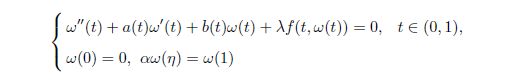# 半正二阶三点边值问题正解的存在性Existence of Positive Solutions Semi-Positive Second-OrderThree-Point Boundary Value Problems

DOI: 10.12677/PM.2021.114083, PDF, HTML, 下载: 23  浏览: 87  国家自然科学基金支持

Abstract: By using the fixed-point theorem in cones, we obtain the existence of positive solutions for the semi-positive second-order three-point boundary value problemswhere λ > 0,  0 < η < 1 satisfies 0 < αη < 1 and f∈C([0,1]×[0,∞),(−∞,∞)), with f(t,ω)≥−M for some positive constants M.

  马如云. 非线性常微分方程非局部问题[M]. 北京: 科学出版社, 2004.  Il’in, V.A. and Moiseev, E.I. (1987) Nonlocal Boundary Value Problem of the First Kind for a Sturm-Liouville Operator in Its Differential and Finite Difference Aspects. Journal of Differential Equations, 23, 803-810.  Gupta, C.P. (1992) Solvability of a Three-Point Nonlinear Boundary Value Problem for a Sec- ond Order Ordinary Differential Equation. Journal of Mathematical Analysis and Applications, 168, 540-551. https://doi.org/10.1016/0022-247X(92)90179-H  Ma, R. (1997) Existence Theorems for a Second Order Three-Point Boundary Value Problem. Journal of Mathematical Analysis and Applications, 212, 430-442. https://doi.org/10.1006/jmaa.1997.5515  Zhang, Q. and Jiang, D. (2010) Multiple Solutions to Semipositone Dirichlet Boundary Value Problems with Singular Dependent Nonlinearities for Second Order Three-Point Differential Equations. Computers and Mathematics with Applications, 59, 2516-2527. https://doi.org/10.1016/j.camwa.2009.12.036  Gao, C., Zhang, F. and Ma, R. (2017) Existence of Positive Solutions of Second-Order Pe- riodic Boundary Value Problems with Sign-Changing Green’s Function. Acta Mathematicae Applicatae Sinica, English Series, 33, 263-268. https://doi.org/10.1007/s10255-017-0657-2  Ma, R., Gao, C. and Chen, R. (2010) Existence of Positive Solutions of Nonlinear Second-Order Periodic Boundary Value Problems. Boundary Value Problems, 2010, Article No. 626054. https://doi.org/10.1155/2010/626054  Ma, R. and Wang, H. (2003) Positive Solutions of Nonlinear Three-Point Boundary-Value Problems. Journal of Mathematical Analysis and Applications, 279, 216-227.https://doi.org/10.1016/S0022-247X(02)00661-3  Yao, Q. (2002) An Existence Theorem of Positive Solution for a Superlinear Semipositone Second-Order Three-Point BVP. Journal of Mathematical Study, 35, 32-35.  姚庆六. 半正二阶三点边值问题的解和正解[J]. 应用数学学报, 2007, 30(2): 209-217.  魏晋洼, 王素云, 李永军. 一类半正二阶常微分方程边值问题正解的存在性[J]. 山东大学学报(理学版), 2019, 54(10): 7-12.  任立页, 安玉坤. 关于半正二阶三点边值问题正解的存在性[J]. 陕西师范大学学报(自然科学版), 2004, 32(3): 31-33.  Guo, D. and Lakshmikantham, V. (1998) Nonlinear Problems in Abstract Cones. Academic Press, San Diego.